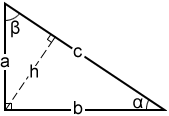# Right Triangle Calculator

Please provide 2 values below to calculate the other values of a right triangle. If radians are selected as the angle unit, it can take values such as pi/3, pi/4, etc.

## Result

Given a=6 and b=28,
c = 28.63564
∠α = 12.095° = 12°5'41" = 0.21109 rad
∠β = 77.905° = 77°54'19" = 1.3597 rad
h = 5.86681
area = 84
perimeter = 62.63564
inradius = 2.68218circumradius = 14.31782+ Show Calculation Steps

 a = ∠α = degree radian b = ∠β = degree radian c = h = A = area P = perimeterRelatedTriangle Calculator | Pythagorean Theorem Calculator

### Right triangle

A right triangle is a type of triangle that has one angle that measures 90°. Right triangles, and the relationships between their sides and angles, are the basis of trigonometry.

In a right triangle, the side that is opposite of the 90° angle is the longest side of the triangle, and is called the hypotenuse. The sides of a right triangle are commonly referred to with the variables a, b, and c, where c is the hypotenuse and a and b are the lengths of the shorter sides. Their angles are also typically referred to using the capitalized letter corresponding to the side length: angle A for side a, angle B for side b, and angle C (for a right triangle this will be 90°) for side c, as shown below. In this calculator, the Greek symbols α (alpha) and β (beta) are used for the unknown angle measures. h refers to the altitude of the triangle, which is the length from the vertex of the right angle of the triangle to the hypotenuse of the triangle. The altitude divides the original triangle into two smaller, similar triangles that are also similar to the original triangle.

If all three sides of a right triangle have lengths that are integers, it is known as a Pythagorean triangle. In a triangle of this type, the lengths of the three sides are collectively known as a Pythagorean triple. Examples include: 3, 4, 5; 5, 12, 13; 8, 15, 17, etc.

Area and perimeter of a right triangle are calculated in the same way as any other triangle. The perimeter is the sum of the three sides of the triangle and the area can be determined using the following equation:

A =
 1 2
ab =
 1 2
ch

### Special Right Triangles

30°-60°-90° triangle:

The 30°-60°-90° refers to the angle measurements in degrees of this type of special right triangle. In this type of right triangle, the sides corresponding to the angles 30°-60°-90° follow a ratio of 1:√3:2. Thus, in this type of triangle, if the length of one side and the side's corresponding angle is known, the length of the other sides can be determined using the above ratio. For example, given that the side corresponding to the 60° angle is 5, let a be the length of the side corresponding to the 30° angle, b be the length of the 60° side, and c be the length of the 90° side.:

Angles: 30°: 60°: 90°

Ratio of sides: 1:√3:2

Side lengths: a:5:c

Then using the known ratios of the sides of this special type of triangle:

a =
 b √3
=
 5 √3
c =
 b × 2 √3
=
 10 √3

As can be seen from the above, knowing just one side of a 30°-60°-90° triangle enables you to determine the length of any of the other sides relatively easily. This type of triangle can be used to evaluate trigonometric functions for multiples of π/6.

45°-45°-90° triangle:

The 45°-45°-90° triangle, also referred to as an isosceles right triangle, since it has two sides of equal lengths, is a right triangle in which the sides corresponding to the angles, 45°-45°-90°, follow a ratio of 1:1:√2. Like the 30°-60°-90° triangle, knowing one side length allows you to determine the lengths of the other sides of a 45°-45°-90° triangle.

Angles: 45°: 45°: 90°

Ratio of sides: 1:1:√2

Side lengths: a:a:c

Given c= 5:

a =
 c √2
=
 5 √2

45°-45°-90° triangles can be used to evaluate trigonometric functions for multiples of π/4.